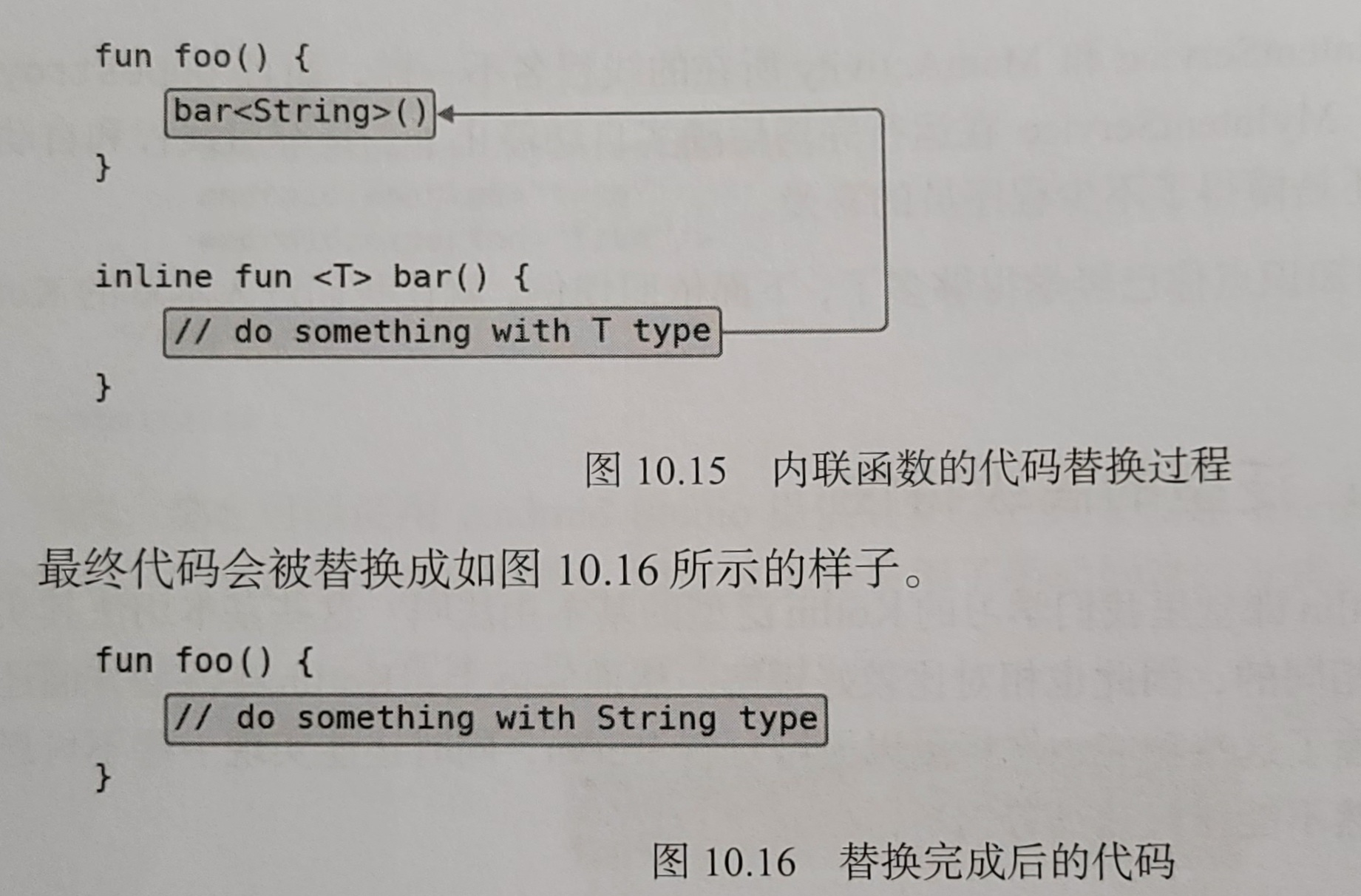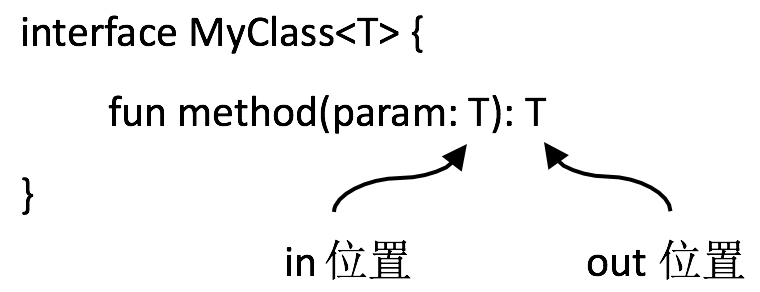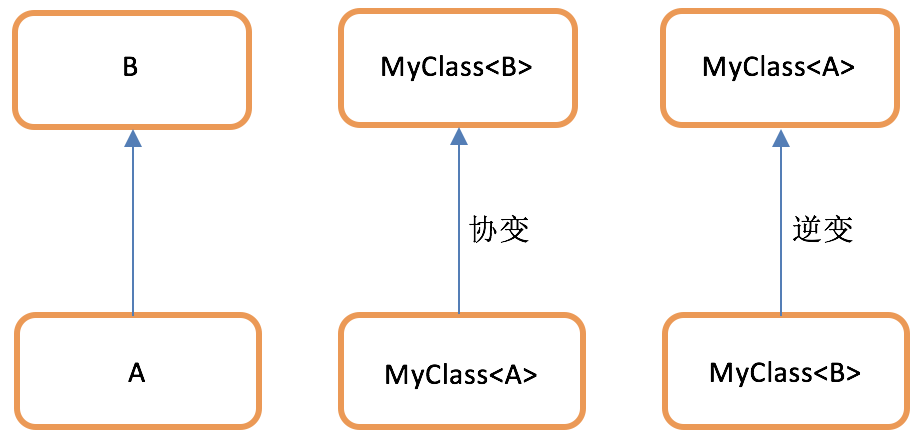## 一、定义泛型类

``````class MyClass<T> {
fun method(param: T): T{
return param
}
}
// 此时的MyClass就是一个泛型类
// MyClass中的方法允许使用T类型的参数和返回值
fun main() {
val myClass = MyClass<Int>()
val result = myClass.method(123)
}
``````

## 二、定义泛型方法

``````class MyClass {
fun <T> method(param: T): T{
return param
}
}

fun main() {
val myClass = MyClass()
val result = myClass.method<Int>(123)
}
``````

## 三、设置泛型上界

``````class MyClass {
// 这个泛型方法只能接收和返回Number类型（Int、Float、Double等）
fun <T : Number> method(param: T): T {
return param
}
}
``````

## 四、利用泛型实现类似apply函数

``````fun <T> T.build(block: T.() -> Unit): T {
block()
return this
}
``````

## 五、对泛型进行实化

### 1.泛型实化原理和简单实现``````// 函数必须是内联函数
// 在声明泛型的地方必须加上reified关键字来表示该泛型要实化
inline fun <reified T> getGenericType() {

}
``````

``````// 只有一行代码，实现了在Java中不可能实现的功能
inline fun <reified T> getGenericType() = T::class.java
``````

``````fun main() {
val result1 = getGenericType<String>()
val result2 = getGenericType<Int>()
println("result1 is \$result1") // result1 is String
println("result2 is \$result2") // result2 is Int
}
``````

### 2.泛型实化的应用

#### 2.1.简化startActivity功能

``````inline fun <reified T> startActivity(context: Context) {
val intent = Intent(context, T::class.java)
context.startActivity(intent)
}

// 使用泛型实化的函数来启动Activity
startActivity<TestActivity>(context)
``````

#### 2.2.简化startActivity功能——带参数

``````inline fun <reified T> startActivity(context: Context,
block: Intent.() -> Unit) {
val intent = Intent(context, T::class.java)
intent.block()
context.startActivity(intet)
}

// 使用泛型实化的函数来带参启动Activity
startActivity<TestActivity>(context) {
putExtra("param1", "data")
putExtra("param2", 123)
}
``````

## 六、泛型的协变和逆变``````open class Person(val name: String, val age: Int)
class Student(name: String, age: Int) : Person(name, age)
class Teacher(name: String, age: Int) : Person(name, age)
``````

### 1.泛型的协变

``````// 泛型类，调用set()和get()方法可以给data字段赋值或读取
class SimpleData<T> {
private var data: T? = null

fun set(t: T?) {
data = t
}

fun get(): T? {
return data
}
}

fun main() {
val student = Student("Tom", 19)
val data = SimpleData<Student>()
data.set(student)
// 假设向接收SimpleData<Person>的方法传入SimpleData<Student>合法
handleSimpleData(data) // 代码会报错，这里假设可以通过编译
// 这里实际会获取到包含Teacher的实例，类型转换异常
val studentData = data.get()
}

fun handleSimpleData(data: SimpleData<Person>) {
val teacher = Teacher("Jack", 35)
// Teacher也是Person子类，给data设置Teache
data.set(teacher)
}
``````

``````// 在泛型T声明前加上out关键字
// 在其泛型类型的数据上只读
// 所有方法都不能接收T类型参数
// 此时不会再有类型转换的安全隐患,SimpleData在泛型T上是协变的
class SimpleData<out T>(val data: T?) {
fun get(): T? {
return data
}
}

fun main() {
val student = Student("Tom", 19)
val data = SimpleData<Student>(student)
handleMyData(data)
// 此时没有任何安全隐患，编译通过
// 同时现在SimpleData<Student>是SimpleData<Person>子类
val studentData = data.get()
}

fun handleMyData(data: SimpleData<Person>) {
val personData = data.get()
}
``````

### 2.@UnsafeVariance注解忽视安全隐患

``````public interface List<out E> : Collection<E> {
override val size: Int
override fun isEmpty(): Boolean
// 原则上协变后泛型E只能出现在out位置上
// 不过contains()目的很明确，它并不会修改当前集合的内容
// 因此这种操作实质上又是安全的，为了让编译器理解这里是安全的
// 可以在泛型前加上@UnsaveVariance注解
override fun contains(element: @UnsaveVariance E): Boolean
override fun iterator(): Iterator<E>
public operator fun get(index: Int): E
}
``````

### 3.泛型的逆变``````// 定义一个Transformer接口，用于执行一些转换操作
// transform()方法接收T类型并返回String类型
interface Transformer<T> {
fun transform(t: T): String
}

// 实现Transformer接口
fun main() {
val trans = object : Transformer<Person> {
override fun transform(t: Person): String {
return "\${t.name} \${t.age}"
}
}
// Transformer<Person>并不是Transformer<Student>子类
handleTransformer(trans) // 这行代码会报错
}

fun handleTransformer(trans: Transformer<Student>) {
val student = Student("Tom", 19)
val result = trans.transform(student)
}
``````

``````// 在T声明前加上in关键字，意味着T只能出现在in位置上
// 同时也意味着Transformer在泛型T上是逆变的
interface Transformer<in T> {
fun transform(t: T): String
}
``````

``````interface Transformer<in T> {
// 让transform()方法接收name和age两个参数
// 由于逆变不允许T出现在out上，所以现在要加上注解
fun transform(name: String, age: Int): @UnsafeVariance T
}

fun main() {
val trans = object : Transformer<Person> {
override fun transform(name: String, age: Int): Person {
// 期望返回Person，实际返回Teacher
// 类型转换异常
return Teacher(name, age)
}
}
handleTransformer(trans)
}

fun handleTransformer(trans: Transformer<String>) {
val result = trans.transform("Tom", 19)
}
``````